Home > CCA2 > Chapter 8 > Lesson 8.1.3 > Problem8-59

8-59.
1. Find the center and radius of each circle below. Homework Help ✎

1. (y − 7)2 = 25 − (x − 3)2

2. x2 + y2 + 10y = −9

3. x2 + y2 + 18x − 8y + 47 = 0

4. y2 + (x − 3)2 = 1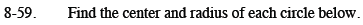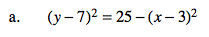Add (x − 3)² to both sides of the equation.

Center: (3, 7)Radius: 5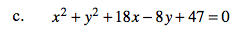See parts (a) and (b).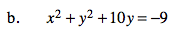Complete the square.

$x^2 + (y^2 +10y + 25) = -9 + 25$

Center: (0, −5) Radius: 4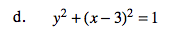See parts (a) and (b).